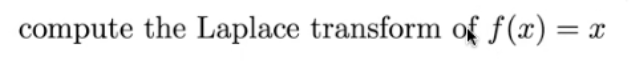# Question compute the Laplace transform of f(x) = =X compute the Laplace transform of f(x) = =X